Learn how to program games with the LÖVE framework

Let's make it so that we can save and load our progress in our game. We do this by writing and reading a file. But first we need to have a game, so let's make a small game where you can pick up coins.

`````` function love.load()
-- Create a player object with an x, y and size
player = {
x = 100,
y = 100,
size = 25
}
end

function love.update(dt)
-- Make it moveable with keyboard
if love.keyboard.isDown("left") then
player.x = player.x - 200 * dt
elseif love.keyboard.isDown("right") then
player.x = player.x + 200 * dt
end

-- Note how I start a new if-statement instead of contuing the elseif
-- I do this because else you wouldn't be able to move diagonally.
if love.keyboard.isDown("up") then
player.y = player.y - 200 * dt
elseif love.keyboard.isDown("down") then
player.y = player.y + 200 * dt
end
end

function love.draw()
-- The players and coins are going to be circles
love.graphics.circle("line", player.x, player.y, player.size)
end``````

And for fun, let's give the player a face.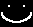`````` function love.load()
-- Create a player object with an x, y and size
player = {
x = 100,
y = 100,
size = 25,
image = love.graphics.newImage("face.png")
}
end

function love.draw()
love.graphics.circle("line", player.x, player.y, player.size)
-- Set the origin of the face to the center of the image
love.graphics.draw(player.image, player.x, player.y,
0, 1, 1, player.image:getWidth()/2, player.image:getHeight()/2)
end``````

Next we want to add some coins. We'll have them positioned randomly on screen. And let's give them a small dollar sign.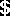`````` function love.load()
player = {
x = 100,
y = 100,
size = 25,
image = love.graphics.newImage("face.png")
}

coins = {}

for i=1,25 do
table.insert(coins,
{
-- Give it a random position with math.random
x = math.random(50, 650),
y = math.random(50, 450),
size = 10,
image = love.graphics.newImage("dollar.png")
}
)
end
end

function love.update(dt)
if love.keyboard.isDown("left") then
player.x = player.x - 200 * dt
elseif love.keyboard.isDown("right") then
player.x = player.x + 200 * dt
end

if love.keyboard.isDown("up") then
player.y = player.y - 200 * dt
elseif love.keyboard.isDown("down") then
player.y = player.y + 200 * dt
end
end

function love.draw()
love.graphics.circle("line", player.x, player.y, player.size)
love.graphics.draw(player.image, player.x, player.y,
0, 1, 1, player.image:getWidth()/2, player.image:getHeight()/2)

for i,v in ipairs(coins) do
love.graphics.circle("line", v.x, v.y, v.size)
love.graphics.draw(v.image, v.x, v.y,
0, 1, 1, v.image:getWidth()/2, v.image:getHeight()/2)
end
end``````

Now we want to be able to pick them up. For that we need to check if there is collision. We do this by calculating the distance, and then checking if the distance is smaller than the radius of both circles summed up.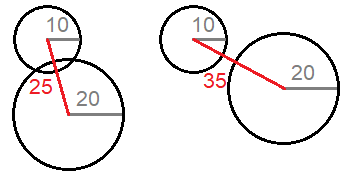``````function checkCollision(p1, p2)
-- Calculating distance in 1 line
-- Subtract the x's and y's, square the difference
-- Sum the squares and find the root of the sum.
local distance = math.sqrt((p1.x - p2.x)^2 + (p1.y - p2.y)^2)
-- Return whether the distance is lower than the sum of the sizes.
return distance < p1.size + p2.size
end``````

And now we iterate through all the coins and check if it's touching the player. Let's make it so that the player grows when picking up a coin.

``````function love.update(dt)
if love.keyboard.isDown("left") then
player.x = player.x - 200 * dt
elseif love.keyboard.isDown("right") then
player.x = player.x + 200 * dt
end

if love.keyboard.isDown("up") then
player.y = player.y - 200 * dt
elseif love.keyboard.isDown("down") then
player.y = player.y + 200 * dt
end

for i,v in ipairs(coins) do
if checkCollision(player, v) then
table.remove(coins, i)
player.size = player.size + 1
end
end
end``````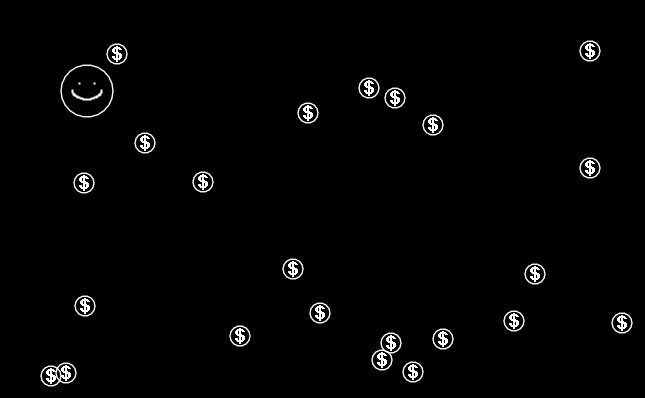Now we can move around and pick up coins. Nice! But before we go over to saving and loading, let's make a few more changes.

If restart the game a few times you might notice that even though the circles are positioned randomly, they are always on the same random spot.

To fix this we can use `math.randomseed()`. The random numbers you generate are based on a number, which we call the seed. And because we don't change the seed you always get the same random positions. The advantage seeds is that they are a key to a certain randomness, which you could save and share. With Minecraft for example, you can share the seed that was used to generate a world, and other people can use it to get the same generated world.

So what number do we use as our seed? Because if we were to do `math.randomseed(123)`, the game would still have the same number. We need a number that is unique every time we start the game. And for that we can use `os.time()`. This is a Lua function that gives you the time of your operation system to the second. That's 86400 unique numbers a day!

But even better might be to use LÖVE's math library. LÖVE's random number generated (rng) is automatically seeded (with os.time()) and overall is better/more random than Lua's rng.

`````` function love.load()
player = {
x = 100,
y = 100,
size = 25,
image = love.graphics.newImage("face.png")
}

coins = {}

for i=1,25 do
table.insert(coins,
{
x = love.math.random(50, 650),
y = love.math.random(50, 450),
size = 10,
image = love.graphics.newImage("dollar.png")
}
)
end
end``````

Another thing that bugs me is the for-loop in which we remove coins. Now, picking up coins works fine, but in general when you are iterating through a list, and you're removing items from that list, you want to use a more safe approach. Because by removing an item, the list gets shorter and it messes up the for-loop.

Try out this code for example:

``````local test = {"a", "b", "c", "d", "e", "f", "g", "h", "i", "j"}
for i,v in ipairs(test) do
table.remove(test, i)
end
print(#test)
-- Output: 5``````

It outputs 5, meaning it only emptied half of the list. This happens because as it removes an item from the list, everything gets moved to the side, and items get skipped over.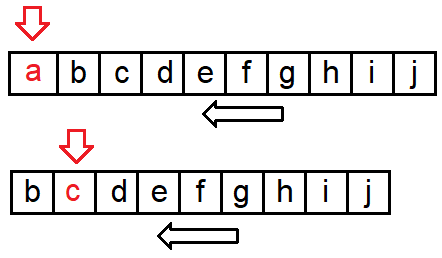To fix this we should loop through the table in reverse. This way you will never skip over any items when you have remove something.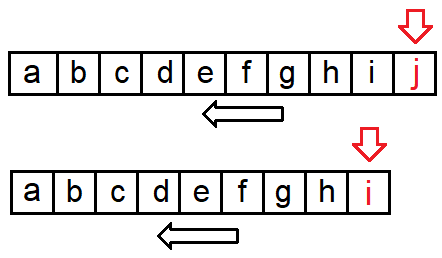This means that we won't be using `ipairs` but instead a normal for-loop, but backwards.

``````function love.update(dt)
if love.keyboard.isDown("left") then
player.x = player.x - 200 * dt
elseif love.keyboard.isDown("right") then
player.x = player.x + 200 * dt
end

if love.keyboard.isDown("up") then
player.y = player.y - 200 * dt
elseif love.keyboard.isDown("down") then
player.y = player.y + 200 * dt
end

-- Start at the end, until 1, with steps of -1
for i=#coins,1,-1 do
-- Use coins[i] instead of v
if checkCollision(player, coins[i]) then
table.remove(coins, i)
end
end
end``````

Alright, time to start saving the game.

## Saving

So what we do for saving the game is that we make a table, and in this table we put all the information that we want to save. So what do we want to save? How about the player's position, his size and the same for the coins that haven't been picked up yet. So let's create the function `saveGame()` where we store the important data in a table.

``````function saveGame()
data = {}
data.player = {
x = player.x,
y = player.y,
size = player.size
}

data.coins = {}
for i,v in ipairs(coins) do
-- In this case data.coins[i] = value is the same as table.insert(data.coins, value )
data.coins[i] = {x = v.x, y = v.y}
end
end``````

So why save these specific parts and not just the whole table? Well in general you don't want to use more data then you need. We don't need to save the image width and height because it will always be the same. Also, our player object has an image object, and we can't save LÖVE objects.

So now that we have all the information we need to serialize it. This means that we need to make the table into something that we can read. Because right now when you print table you might get something like `table: 0x00e4ca20`, and that's not the information we want to save.

To serialize the table we're going to use lume which is a utility library by rxi. You can find the library on GitHub.

Click on `lume.lua` and then on Raw, and copy the code.

Go to your text editor, create a new file called `lume.lua` and paste the code. Load it with `require` in `main.lua` at the top of `love.load()`.

Lume has all kinds of neat functions, but the important ones for this is tutorial are `serialize` and `deserialize`. Let's try it out. Serialize the data table and then print its value.

``````function saveGame()
data = {}
data.player = {
x = player.x,
y = player.y,
size = player.size
}

data.coins = {}
for i,v in ipairs(coins) do
-- In this case data.coins[i] = value is the same as table.insert(data.coins, value )
data.coins[i] = {x = v.x, y = v.y}
end

serialized = lume.serialize(data)
print(serialized)
end``````

In your output you'll see the table printed in a way that you can read it. This is what we'll be saving to our file, which is the next step.

We can create, edit and read files with the `love.filesystem` (wiki) module. For create/writing a file we use `love.filesystem.write(filename, data)` (wiki). The first argument is the name of the file, the second argument is the data that we want to write to the file.

``````function saveGame()
data = {}
data.player = {
x = player.x,
y = player.y,
size = player.size
}

data.coins = {}
for i,v in ipairs(coins) do
-- In this case data.coins[i] = value is the same as table.insert(data.coins, value )
data.coins[i] = {x = v.x, y = v.y}
end

serialized = lume.serialize(data)
-- The filetype actually doesn't matter, and can even be omitted.
love.filesystem.write("savedata.txt", serialized)
end``````

Now we need to make that you can save. Let's make it so that you save when you press f1.

``````function love.keypressed(key)
if key == "f1" then
saveGame()
end
end``````

Run the game, grab some coins and press F1. Just like that we made our first save file! So where is it? If you're on Windows it's saved in `AppData\Roaming\LOVE\`. You can get to the hidden AppData folder by pressing Ctrl + R, followed by typing "appdata" and click on OK. There should be a folder that has the same name as the folder your LÖVE project is in. And in that folder you will find a file named `savedata.txt`. If you open the file you'll see that you're table is inside.

Now let's make it so that we can load our data.

To load our data we need to:

• Check if a save file exists
• Turn the data into a table
• Apply the data to our players and coins.

So let's start with checking if our file exists, and if so we read the file. We can do this with `love.filesystem.getInfo(filename)` and `love.filesystem.read(filename)`. If a file exists, `love.filesystem.getInfo(filename)` will return a table with information, else it will return `nil`. Since we only want to know if the file exists we can put the function in an if-statement, because we don't need to the information that the table provides.

`````` function love.load()

lume = require "lume"

player = {
x = 100,
y = 100,
size = 25,
image = love.graphics.newImage("face.png")
}

coins = {}

if love.filesystem.getInfo("savedata.txt") then
print(file)
end

for i=1,25 do
table.insert(coins,
{
x = love.math.random(50, 650),
y = love.math.random(50, 450),
size = 10,
image = love.graphics.newImage("dollar.png")
}
)
end
end``````

Run the game and it should print our save data. Now we need to turn this table string into a real table. We can do this with `lume.deserialize`.

``````if love.filesystem.getInfo("savedata.txt") then
data = lume.deserialize(file)
end``````

And now we can apply the data to our player and coins. Now how we put this code before filling in the coins table. That's because we don't want generate the coins that we have already picked up in our save file. Which coins we add is now based on the data.

`````` function love.load()

lume = require "lume"

player = {
x = 100,
y = 100,
size = 25,
image = love.graphics.newImage("face.png")
}

coins = {}

if love.filesystem.getInfo("savedata.txt") then
data = lume.deserialize(file)

--Apply the player info
player.x = data.player.x
player.y = data.player.y
player.size = data.player.size

for i,v in ipairs(data.coins) do
coins[i] = {
x = v.x,
y = v.y,
size = 10,
image = love.graphics.newImage("dollar.png")
}
end
else
-- Only execute this if you don't have a save file
for i=1,25 do
table.insert(coins,
{
x = love.math.random(50, 650),
y = love.math.random(50, 450),
size = 10,
image = love.graphics.newImage("dollar.png")
}
)
end
end
end``````

Now when you run the game you'll see that it loads your save file. You can grab some more coins, press F1 to save, and when you restart the game you'll see that it again has saved and loaded your game. Awesome! But what if we want to restart? Let's add a button that deletes our save file so that we can start a new game.

## Resetting

To remove our save file we can use `love.filesystem.remove(filename)`. Let's make it so that when we press F2 the file is removed and it restarts the game. We can quit the game with `love.event.quit()`, but if we pass `"restart"` as first argument, the game will restart instead.

``````function love.keypressed(key)
if key == "f1" then
saveGame()
elseif key == "f2" then
love.filesystem.remove("savedata.txt")
love.event.quit("restart")
end
end``````

And there we go, we can now reset our game!

## Summary

Seeds decide which random values you generate, and this can be used to share a randomly generated level for example. We can also use LÖVE's math module. When removing items from a list we should loop through the table in reverse to prevent items from being skipped. We can create a save file by adding important data to a table, then turn that table into a string and write that string to a file with `love.filesystem.write(filename)`. We can load a save file by reading the file with `love.filesystem.read(filename)`, deserializing the data and applying the data to our objects. We can remove a file with `love.filesystem.remove(filename)` and restart the game with `love.event.quit("restart")`.

Do you need help or do you see a mistake?